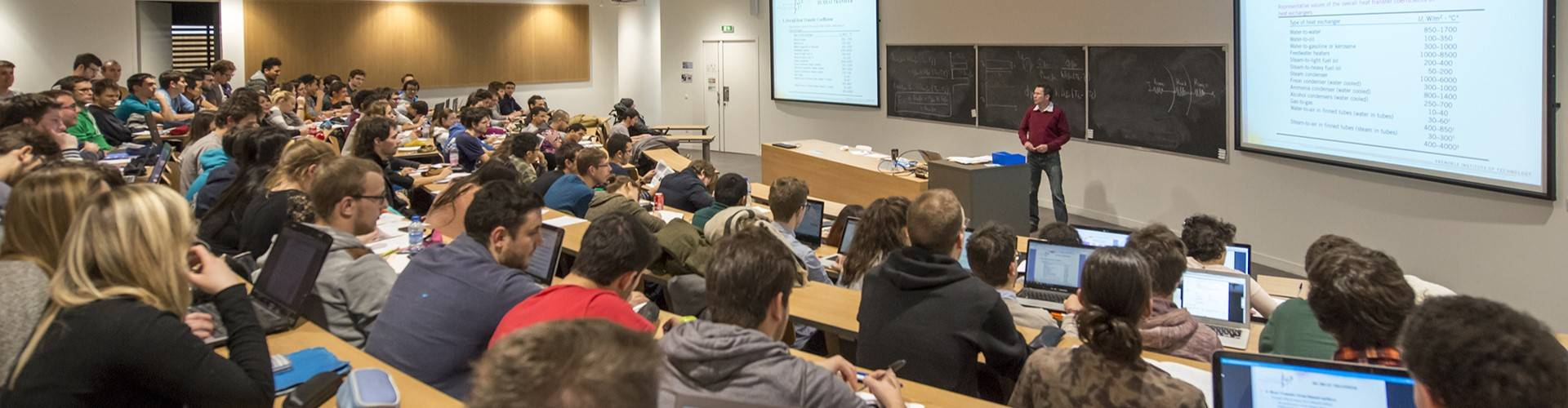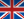# Artificial Intelligence and Autonomous Systems (M2-MARS / ASI) - WEUMAIA0

• #### Number of hours

• Lectures 32.0
• Projects -
• Tutorials -
• Internship -
• Laboratory works 28.0
• Written tests -

ECTS 5.0

## Goal(s)

Introduction to MACHINE and STATISTICAL LEARNING (30 hours, including courses and lab work)
Introduction to AI FOR AUTONOMOUS SYSTEMS (30 hours, including courses and lab work)

Responsible(s)

Olivier MICHEL

## Content(s)

MACHINE LEARNING and Statistical LEARNING PRINCIPLES (ML)
This part of the course aims at providing the students with the basic methods and statistical/mathematical tools to understand the most classical approaches used in both supervised or unsupervised learning, as well as understanding their capabilities, characteristics and limitations.

ML-1: "Introduction to Machine Learning
- motivations
- basic challenges: classification/regression, Supervised/unsupervised, cost functions, training error/testing
- examples of approaches WITH and WITHOUT models
- Notion of complexity: variance bias trade-off (over or under learning), scourge of the dimension

ML-2: Generative Approaches :
- Discriminant analysis (linear/quadratic, regularization, size reduction)
- Naive Bayesian (parametric and non-parametric
- examples given MNIST, ...

ML-3: Discriminatory approaches :
- generalized linear statistical models for regression and classification (logistic regression)
- ridge regulation, lasso (parsimony bet)
- Complexity and validation (cross-validation) of models
- Data transformation (kernel move)
- Examples given MNIST, ...

ML-4: "DATA REPRESENTATION" (Unsupervised).
- BCP, standardization, small visualization

ML-5. "(Convolutional) Neural Nets."
- Deep, convolutional aspects... motivations and examples (scikit-learn, kheras/tensorflow,...)

ML-6. Random trees and forests
- decision tree, random forests

ML-7. "CLUSTERING
- Kmeans, Gaussian mixture model, EM algorithm,
- Ex MNIST data and comparison with CNN
- hierarchical patterns

Prerequisites

AI for Autonomous systems

ML :
probabilities and statistics, main distributions, probability density functions, matrix calculation, basic optimization (Newton's methods, Gradient methods...)
The math reminders will be done in general (the prerequisites having been seen in 1A and 2A), but very quickly.

Test

AI prt (50%)
ML part (50%): 1h short written exam (25%), ML mini-project (25%)

AI (50%)
ML(50%)

The exam is given in english onlyCalendar

The course exists in the following branches:

see the course schedule for 2023-2024

Course ID : WEUMAIA0
Course language(s):You can find this course among all other courses.

Bibliography

AI :
********

ML :

Trevor Hastie, Robert Tibshirani et Jerome Friedman (2009), "The Elements of Statistical Learning," (2nd Edition) Springer Series in Statistics

Christopher M. Bishop (2006), "Pattern Recognition and Machine Learning," Springer

Kevin P. Murphy (2012), "Machine Learning: a Probabilistic Perspective", The MIT Press